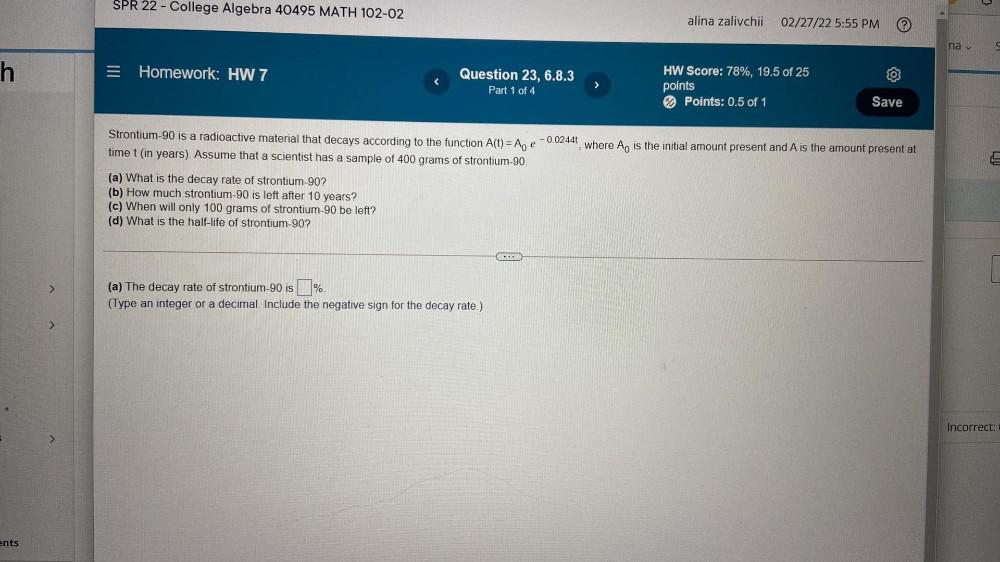Question:

# SPR 22 - College Algebra 40495 MATH 102-02 alina zalivchii 02/27/22 5:55 PM na v h = Homework: HW 7 Question 23, 6.8.3 Part 1 ofSPR 22 - College Algebra 40495 MATH 102-02 alina zalivchii 02/27/22 5:55 PM na v h = Homework: HW 7 Question 23, 6.8.3 Part 1 of 4 HW Score: 78%, 19.5 of 25 points Points: 0.5 of 1 Save Strontium-90 is a radioactive material that decays according to the function A(t) = A time t (in years). Assume that a scientist has a sample of 400 grams of strontium-90. -0.0244 e where Ao is the initial amount present and A is the amount present at (a) What is the decay rate of strontium-90? (b) How much strontium-90 is left after 10 years? (c) When will only 100 grams of strontium-90 be left? (d) What is the half-life of strontium-90? (a) The decay rate of strontium-90 is % (Type an integer or a decimal. Include the negative sign for the decay rate.) Incorrect: > ents